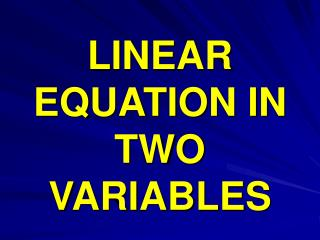DownloadDownload PresentationLINEAR EQUATION IN TWO VARIABLES

# LINEAR EQUATION IN TWO VARIABLES

Download Presentation## LINEAR EQUATION IN TWO VARIABLES

- - - - - - - - - - - - - - - - - - - - - - - - - - - E N D - - - - - - - - - - - - - - - - - - - - - - - - - - -
##### Presentation Transcript

1. LINEAR EQUATION IN TWO VARIABLES

2. System of equations or simultaneous equations – A pair of linear equations in two variables is said to form a system of simultaneous linear equations. For Example, 2x – 3y + 4 = 0 x + 7y – 1 = 0 Form a system of two linear equations in variables x and y.

3. The general form of a linear equation in two variables x and y is ax + by + c = 0 , a =/= 0, b=/=0, where a, b and c being real numbers. A solution of such an equation is a pair of values, one for x and the other for y, which makes two sides of the equation equal. Every linear equation in two variables has infinitely many solutions which can be represented on a certain line.

4. GRAPHICAL SOLUTIONS OF A LINEAR EQUATION • Let us consider the following system of two simultaneous linear equations in two variable. • 2x – y = -1 • 3x + 2y = 9 Here we assign any value to one of the two variables and then determine the value of the other variable from the given equation.

5. X 0 2 Y 1 5 For the equation 2x –y = -1 ---(1) 2x +1 = y Y = 2x + 1 3x + 2y = 9 --- (2) 2y = 9 – 3x 9- 3x Y = ------- 2 X 3 -1 Y 0 6

6. Y (-1,6) (2,5) (0,3) (0,1) X X’ X= 1 Y=3 Y’

7. ALGEBRAIC METHODS OF SOLVING SIMULTANEOUS LINEAR EQUATIONS The most commonly used algebraic methods of solving simultaneous linear equations in two variables are Method of elimination by substitution Method of elimination by equating the coefficient Method of Cross- multiplication

8. ELIMINATION BY SUBSTITUTION STEPS Obtain the two equations. Let the equations be a1x + b1y + c1 = 0 ----------- (i) a2x + b2y + c2 = 0 ----------- (ii) Choose either of the two equations, say (i) and find the value of one variable , say ‘y’ in terms of x Substitute the value of y, obtained in the previous step in equation (ii) to get an equation in x

9. ELIMINATION BY SUBSTITUTION Solve the equation obtained in the previous step to get the value of x. Substitute the value of x and get the value of y. Let us take an example x + 2y = -1 ------------------ (i) 2x – 3y = 12 -----------------(ii)

10. SUBSTITUTION METHOD x + 2y = -1 x = -2y -1 ------- (iii) Substituting the value of x in equation (ii), we get 2x – 3y = 12 2 ( -2y – 1) – 3y = 12 - 4y – 2 – 3y = 12 - 7y = 14 , y = -2 ,

11. SUBSTITUTION Putting the value of y in eq (iii), we get x = - 2y -1 x = - 2 x (-2) – 1 = 4 – 1 = 3 Hence the solution of the equation is ( 3, - 2 )

12. ELIMINATION METHOD • In this method, we eliminate one of the two variables to obtain an equation in one variable which can easily be solved. Putting the value of this variable in any of the given equations, the value of the other variable can be obtained. • For example: we want to solve, 3x + 2y = 11 2x + 3y = 4

13. Let 3x + 2y = 11 --------- (i) 2x + 3y = 4 ---------(ii) Multiply 3 in equation (i) and 2 in equation (ii) and subtracting eq iv from iii, we get 9x + 6y = 33 ------ (iii) 4x + 6y = 8 ------- (iv) 5x = 25 => x = 5

14. putting the value of y in equation (ii) we get, 2x + 3y = 4 2 x 5 + 3y = 4 10 + 3y = 4 3y = 4 – 10 3y = - 6 y = - 2 Hence, x = 5 and y = -2

15. Prepared by :- Mr. Kartik Chandra Behera, Asst. teacher, Govt. Boys’ High School, Unit - IX, Bhubaneswar

16. THANK YOU